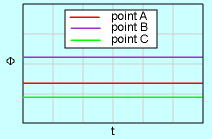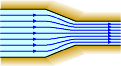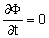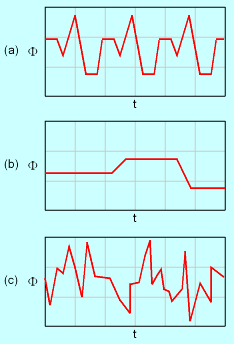Ch 3. Fluid Kinematics Multimedia Engineering Fluids FlowDescriptions Steady &Unsteady Streamlines,Streaklines Velocity &Acceleration IrrotationalFlow
 Chapter 1. Basics 2. Fluid Statics 3. Kinematics 4. Laws (Integral) 5. Laws (Diff.) 6. Modeling/Similitude 7. Inviscid 8. Viscous 9. External Flow 10. Open-Channel Appendix Basic Math Units Basic Equations Water/Air Tables Sections Search eBooks Dynamics Fluids Math Mechanics Statics Thermodynamics Author(s): Chean Chin Ngo Kurt Gramoll ©Kurt GramollFLUID MECHANICS - THEORY In this section, the discussion is on the difference between steady and unsteady flow. Steady Versus Unsteady FlowSteady FlowSteady Flow with Changing Velocity For steady flow, all fluid flow properties (e.g., velocity, temperature, pressure, and density) are independent of time. That is,where Φ represents a fluid property. The properties, however, may vary from point to point, which means that they could be a function of space (i.e., T = T(x, y, z), p = p(x, y, z) and ρ = ρ(x, y, z)). In the study of fluid mechanics it is often assumed that the flow is steady to simplify the analysis but yet give a realistic representation of the real flow field. This is particularly true of basic books on Fluid mechanics, such as this eBook. On the other hand, most flows encountered in real world applications are unsteady flows. It should be noted, steady flow does not mean the velocity and accelerations are constant. Flow in a curved pipe or through a nozzle may be steady, but the velocity and/or acceleration is not constant. This is a common misconception based in part on particle dynamics for rigid bodies.Unsteady Flow: (a) Periodic Flow, (b) Non-Periodic Flow and (c) Random Flow For unsteady flow, the fluid properties are function of time (i.e., T = T(x, y, z, t), p = p(x, y, z, t) and ρ = ρ(x, y, z, t)). Unsteady flows can be further divided into periodic flow, nonperiodic flow and random flow. The graphical representations of these flows are given in the figure. For periodic flow, the property change is repeated in a predictable manner whereas the fluid motion and properties are difficult to predict in random flow as in turbulent flow.

Practice Homework and Test problems now available in the 'Eng Fluids' mobile app
Includes over 250 problems with complete detailed solutions.
Available now at the Google Play Store and Apple App Store.